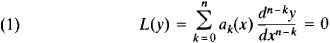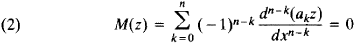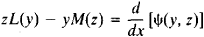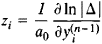The following article is from The Great Soviet Encyclopedia (1979). It might be outdated or ideologically biased.

a concept of the theory of differential equations. The adjoint of the differential equationis the differential equationThe relation is symmetric: L(y) = 0 is also the adjoint of M(z) = 0.

Adjoint differential equations satisfy the identityThe expression ψ(y, z) is known as the bilinear concomitant. It is linear in y and z and in the derivatives of y and z through the (n – 1)st order. If we know k integrals of the adjoint equation, we can decrease the order of the original equation by k.

If

(3) y1, y2, ..., yn

is a fundamental system of solutions of equation (1), then the fundamental system of solutions of equation (2) is given by the formulaswhere i = 1, 2, ... , n and Δ is the Wronskian of system (3). If boundary conditions are given for equation (1), then there exist for equation (2) adjoint boundary conditions such that equation (1), equation (2), and the corresponding boundary conditions determine adjoint differential operators.

The concept of adjoint differential equations can be extended to systems of differential equations and to partial differential equations.